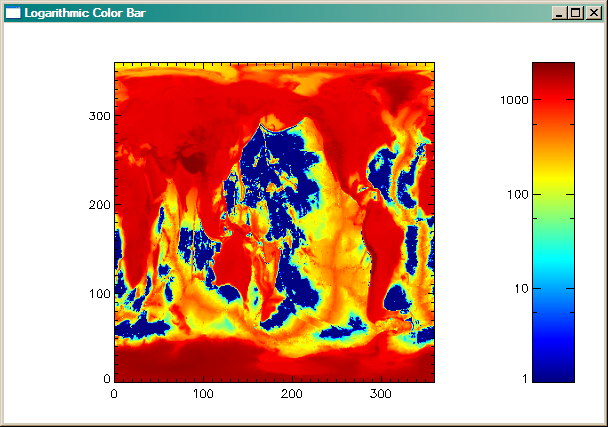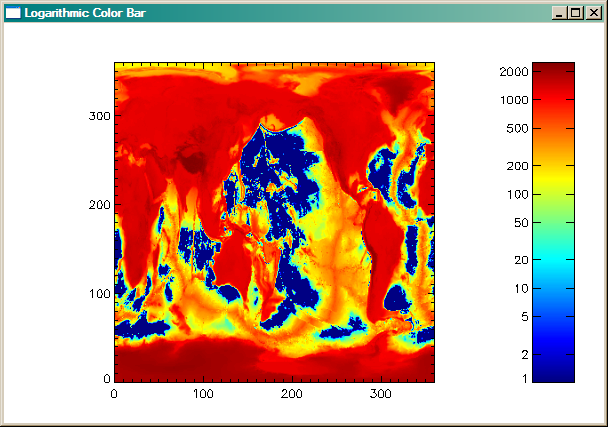# Displaying Logarithmic Color Bar with Data

QUESTION: I have a 2D data set that is scaled from 1 to 2500. How can I display this data as an image with a logarithmic color bar next to it?ANSWER: As long as you are talking about logarithmic scaling to the base 10 (i.e, you are using the IDL routine ALOG10 for scaling), the answer is fairly simple. You can use the cgColorbar routine from the Coyote Library to do this for you.

Consider an example data set scaled in the manor you describe.

```   image = cgDemoData(7) ; World Elevation Data
image = cgScaleVector(image, 1, 2500)
```

To display this data with a logarithmic color bar, you will need to set two additional keywords in the cgColorbar program: YLOG and YTICKS. Note that these keywords are not defined for cgColorbar, but are "extra" keywords that are passed along to the cgPlot command inside cgColorbar. The cgPlot command is used internally to draw the color bar outlines. Also note that if you were displaying a horizontal color bar, these two keywords would be replaced with XLOG and XTICKS The code looks like this.

```   s = Size(image, /Dimensions)
cgDisplay, 600, 400, Title='Logarithmic Color Bar'
pos = [0.1, 0.1, 0.8, 0.9]
cgImage, BytScl(ALog10(image)), Position=pos, /Keep_Aspect, \$
XRange=[0,s], YRange=[0,s], /Axes
cgColorbar, /YLOG, YTICKS=0, Range=[Min(image), Max(image)], /Vertical
```An image displayed with a logarithmic color bar.

Note that if you want the log axis of the color bar to be labeled differently, you could use the LogLevels program, described in this article. Simply replace the cgColorbar command above, with this command:

```   cgColorbar, /YLOG, Range=[Min(image), Max(image)], \$
/Vertical, YTICKS=10, YMINOR=0, YTICKV=LogLevels([1,2500], /Fine)
```

You see the results in the figure below.An image displayed with a more detailed logarithmic color bar.Web Coyote's Guide to IDL Programming